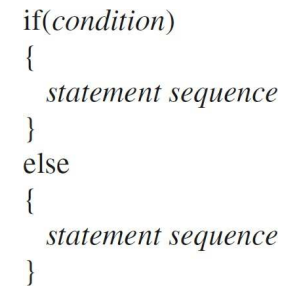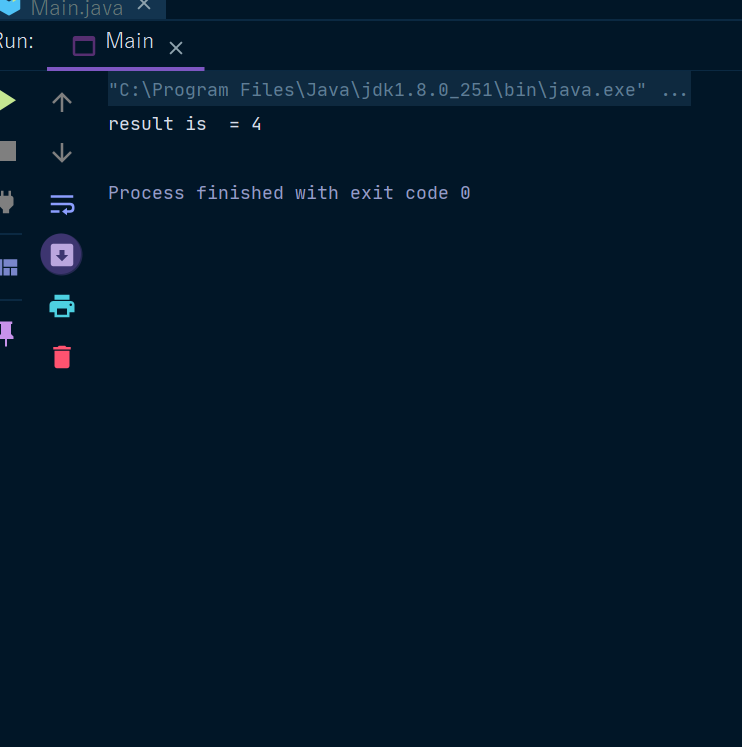Java If Keyword

# Java If Keyword

If Keyword In Java
The if-else statement is the most basic way to control program flow. The else is optional.
If statement executes when the condition is true, In other words-
If the conditional expression is true, the target of the if will be executed; otherwise, if it exists, the target of the else will be executed. At no time will both of them be executed. The conditional expression controlling the if must produce a boolean result.
The Syntax of the if keyword is as follows:In java we have 4 conditional statements -
1.  if
2. else
4. Switch-case
Now we will see the usage with the help of examples -
Example 1 - In this example we will see the usage of if only-
 class T{ public static void main(System.in) { String a = "Web designing house"; String b = "Web designing house" if(a.equals(b)) System.out.println("a and b are same"); } }
 class T{ public static void main(System.in) { String a = "Web designing house"; String b = "Web designing house" if(a.equals(b)) System.out.println("a and b are same"); } }

Example 2 -

 class T{ public static void main(System.in) { String a = "Online promotion house"; String b = "Web designing house" if(a.equals(b)) System.out.println("a and b are same"); else System.out.println("a, and b are different"); } }

Example 3 -

 package javaLearnings; public class Main {   public static void main(String[] args) {   // write your code here               int a =100;               int b =23;           if (a==0){               System.out.println("divide can happen");           }         else if (b==0){             System.out.println("divide can not happen please change value of b");           }               else{           int res = a / b;           System.out.println("result is  = "+res);       }   } }

Output -